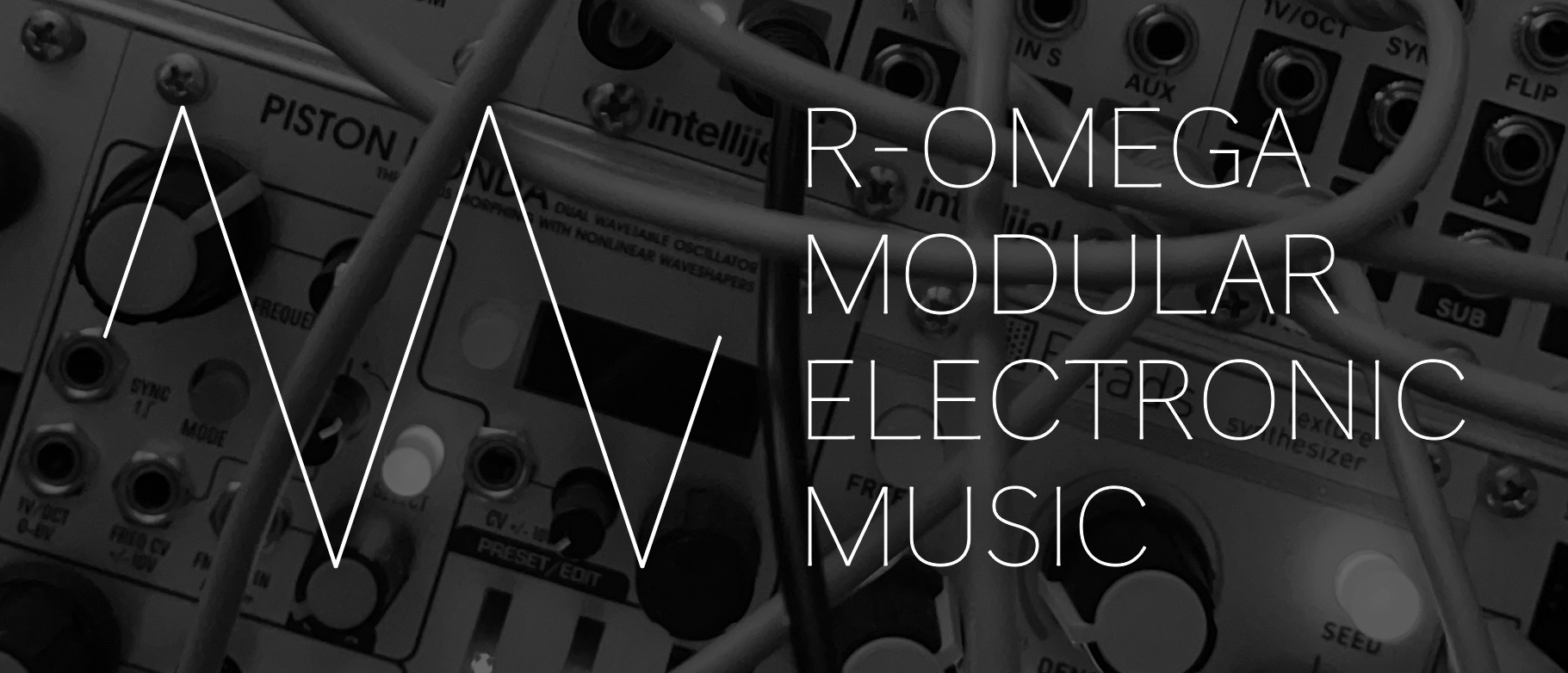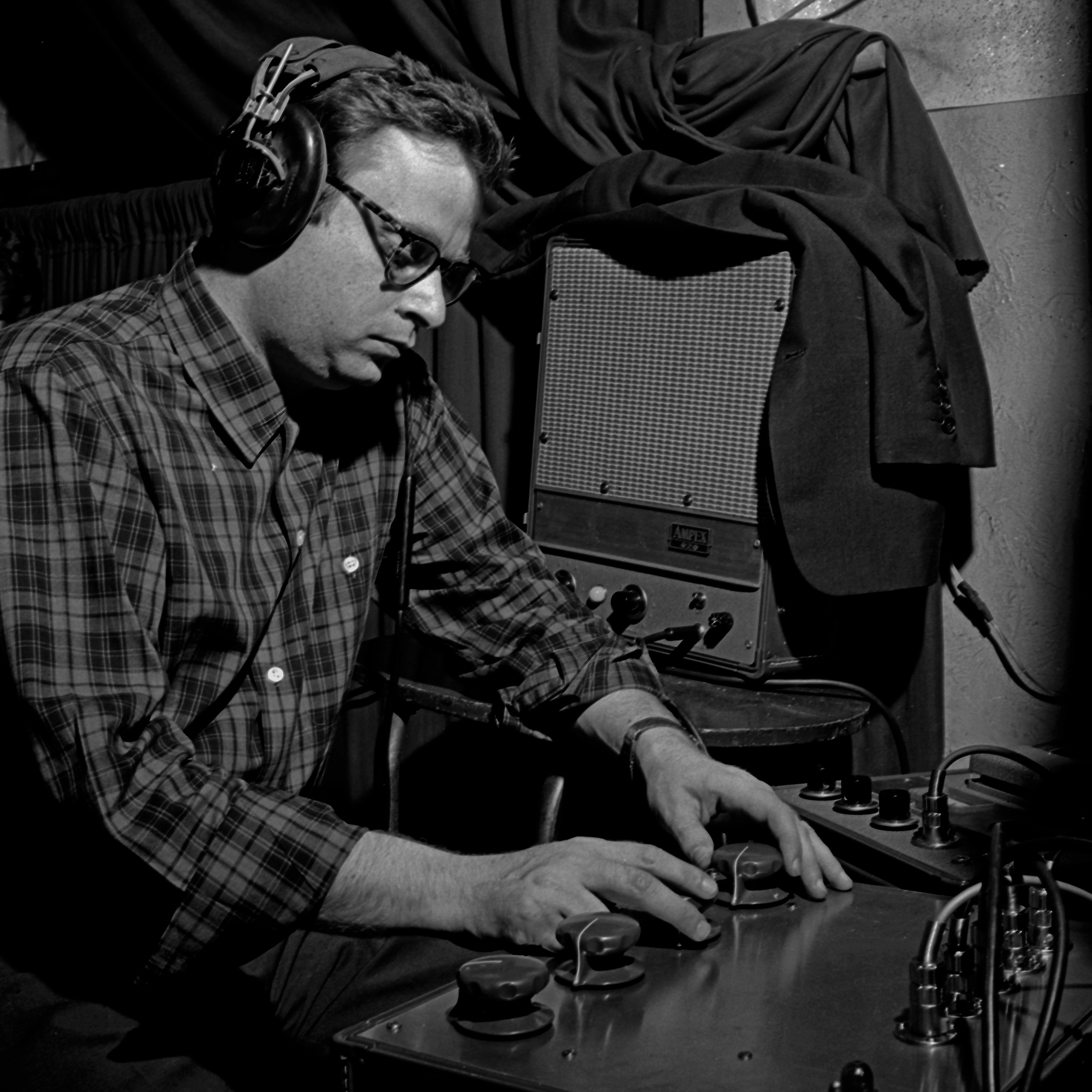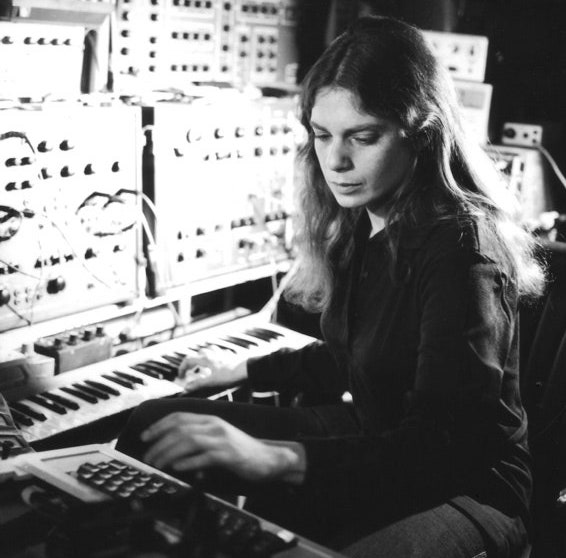Rω (pronounced "R-Omega") is an infinite-dimensional space residing at the boundaries of our intellect. We first learn of R2 in middle school (two-dimensional space), and our physical universe can be described in terms of R4 (four-dimensional spacetime). Rω, however, is an infinite-dimensional space even larger than R ("R-Infinity").

Any point in any space can be described by a sequence of real numbers called a tuple, each number being an ordinate (points in R2 are described by 2-tuples, nicknamed coordinates). Rω is just like R in the sense that its tuples are infinite sequences of real numbers. But while every infinite tuple in R must eventually end with an infinite string of zeros, each of the infinite ordinates in an Rω tuple is free to be any real number.

One can think of R2 as being contained inside R3 if a zero ordinate is annexed to each coordinate in R2 (geometrically, we can envision the two-dimensional Euclidean plane of R2 resting inside the three-dimensional space of R3). Similarly, every n-dimensional space Rn is contained within R if an infinite string of zeros is annexed to each n-tuple. What this means, then, is R is actually contained inside the much larger Rω.

## Works

Click the icon in the upper right of the window to select a video or just press play

## Inspiration## Piston Honda Warm & Smooth Wavetable Banks

Below is a link to a zip file with custom wavetable banks for the Piston Honda module.